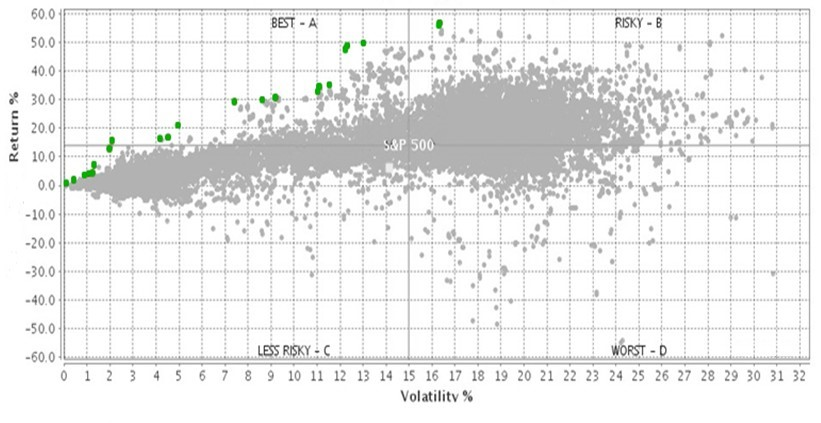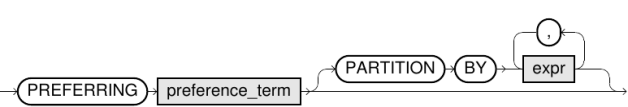# Skyline

Skyline is an SQL extension for data analysis developed by Exasol. It solves the following problems with large data volumes with multi-criteria optimization:

• Data flooding: Large data volumes can hardly be analyzed. When navigating through millions of data rows, you typically sort by one dimension and regard the top N results. By that, you will nearly always miss the optimum result.
• Empty result: You often use filters to simplify the problem of large data volumes. Most of the time, they are too restrictive and lead to empty result sets. By iteratively adjusting the filters, people try to extract a controllable data set. This procedure prevents finding the optimum result.
• The difficulty of correlating many dimensions: When many dimensions are relevant, you can hardly find an optimum result through regular SQL. By the use of metrics, analysts try to find an adequate heuristic to weight the different attributes. But by simplifying the problem to only one single number, a lot of information and correlation within the data is eliminated. The optimum result may not be possible by this strategy.

## How Skyline Works

Instead of using hard filters through the WHERE clause and metrics between columns, the relevant dimensions are specified in the PREFERRING clause. Skyline uses this to provide the optimal set. The optimal set, also known as the Pareto set, which is the number of non-dominated points in the search space. By definition, a point is dominated by another one if it is inferior or equal in all dimensions, and it must be inferior in at least one dimension.

Let's take a scenario of optimized space with just two dimensions for better understanding. You have to decide on a car by using two attributes - high power and low price. A car A is dominated by car B if its price is lower or equal, and its performance is higher or equal than A, but they are not equal in both dimensions. It will be challenging to decide if only one dimension is superior and the other dimension is inferior. In this case, the Pareto set will be the set of cars with preferably high performance and low price.

Without Skyline, you can only try to find a reasonable metric by combining the performance and price in a formula or limiting the results by a certain price or performance range. However, the optimum result won't be identified by this approach.

With Skyline, you can specify the two dimensions within the PREFERRING clause (PREFERRING HIGH power PLUS LOW price) and get the best result in return. The Skyline algorithm compares all the rows of a table with each other based on the PREFERRING clause. In contrast, you can determine (sorting by number) the result by using a simple metric. However, the complexity of the correlations is totally eliminated. Skyline provides the possibility of considering the structure of the data and finding the optimum result set.

You can use the PREFERRING clause in the SELECT, DELETE and UPDATE statements.

## Example

To illustrate the capabilities of Skyline, let's consider the selection of the best funds in the market. To select good funds, you can use attributes, such as performance, volatility, an investment fee, ratings, yearly cost, and so on. To simplify the example, let's take the first two attributes (performance and volatility). Performance and volatility have a reversed correlation. The more conservative a fund is, the lower its volatility is, and so is performance.``SELECT * FROM funds PREFERRING HIGH performance PLUS LOW volatility;``

In the above image, thousands of funds are plotted based on its performance and volatility. The green dots are the results provided by the Skyline query. These funds represent the optimum subset based on the two dimensions. Based on this result, you can decide on the best fund in the market.

## Syntax Elements

As described in the statement SELECT, you can define the PREFERRING clause after the WHERE condition. It contains the following elements:

preferring_clause::=preference_term::=Element Description

PARTITION BY

If you specify this option, then the preferences are evaluated separately for each partition.

HIGH and LOW

Defines whether an expression should have high or low values. Please note that numerical expressions are expected here.

Boolean expressions

In the case of Boolean expressions, the elements are preferred where the condition results in TRUE. The expression x>0 is, therefore, equivalent to HIGH (x>0). The latter expression would be implicitly converted into the numbers 0 and 1.

PLUS

Through the keyword PLUS, multiple expressions of the same importance can be specified.

PRIOR TO

With this clause, you can nest two expressions hierarchically. The second term will only be considered if two elements have the same value for the first term.

INVERSE

By using the keyword INVERSE, you can create the opposite / inverse preference expression. Therefore, the expression LOW price is equivalent to INVERSE(HIGH price).

The following example shows a more complex scenario to select a car based on the nested expressions:

• The performance is segmented by steps of tens.
• The price is segmented by steps of thousands.
• In the case of identical performance and price, the color silver is preferred.
``SELECT * FROM cars    PREFERRING (LOW ROUND(price/1000) PLUS HIGH ROUND(power/10))        PRIOR TO (color = 'silver');``